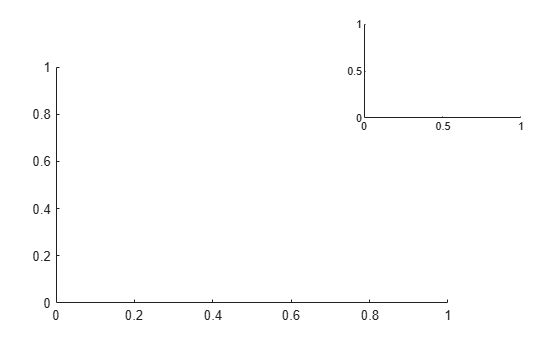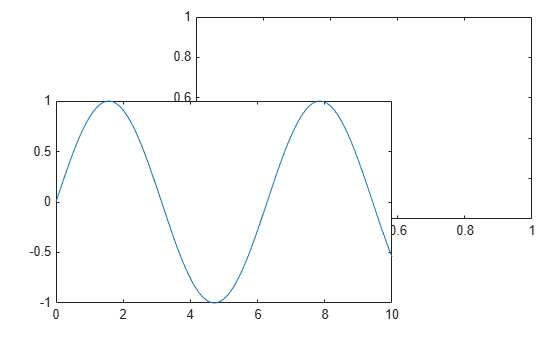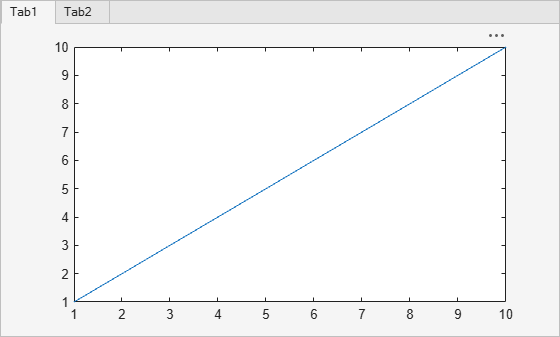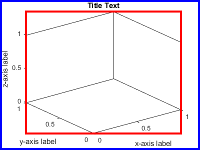Main Content

# axes

Create Cartesian axes

## Syntax

``axes``
``axes(Name,Value)``
``axes(parent,Name,Value)``
``ax = axes(___)``
``axes(cax)``

## Description

````axes` creates the default Cartesian axes in the current figure and makes it the current axes. Typically, you do not need to create axes before plotting since graphics functions automatically create axes when plotting if they do not exist.```

example

````axes(Name,Value)` modifies the axes appearance or controls the way data displays using one or more name-value pair arguments. For example, `'FontSize',14` sets the font size for the axes text. For a list of properties, see Axes Properties.```

example

````axes(parent,Name,Value)` creates the axes in the figure, panel, or tab specified by `parent`, instead of in the current figure.```
````ax = axes(___)` returns the `Axes` object created. Use `ax` to query and modify properties of the `Axes` object after it is created. For a list of properties, see Axes Properties.```
````axes(cax)` makes the axes or standalone visualization specified by `cax` the current axes and brings the parent figure into focus. This command also makes `cax` the first object listed in the `Children` property of the figure and sets the `CurrentAxes` property of the figure to `cax`.```

## Examples

collapse all

Position two `Axes` objects in a figure and add a plot to each one.

Specify the position of the first `Axes` object so that it has a lower left corner at the point (0.1 0.1) with a width and height of 0.7. Specify the position of the second `Axes` object so that it has a lower left corner at the point (0.65 0.65) with a width and height of 0.28. By default, the values are normalized to the figure. Return the `Axes` objects as `ax1` and `ax2`.

```figure ax1 = axes('Position',[0.1 0.1 0.7 0.7]); ax2 = axes('Position',[0.65 0.65 0.28 0.28]);```Add a plot to each `Axes` object. Specify the axes by passing it as the first input argument to the graphics function. Most graphics functions reset some axes properties, such as the tick values and labels. However, they do not reset the axes position.

```contour(ax1,peaks(20)) surf(ax2,peaks(20))```Create two overlayed `Axes` objects. Then, specify the current axes and add a plot.

First create two `Axes` objects and specify the positions. Display the box outline around each axes. Return the `Axes` objects as `ax1` and `ax2`.

```figure ax1 = axes('Position',[0.1 0.1 .6 .6],'Box','on'); ax2 = axes('Position',[.35 .35 .6 .6],'Box','on');```Make `ax1` the current axes. This action brings the axes to the front of the display and makes it the target for subsequent graphics functions. Add a line plot to the axes.

```axes(ax1) x = linspace(0,10); y = sin(x); plot(x,y)```Create a figure with two tabs. Add axes to each tab by specifying the parent container for each one. Plot a line in the first tab and a surface in the second tab.

```figure tab1 = uitab('Title','Tab1'); ax1 = axes(tab1); plot(ax1,1:10) tab2 = uitab('Title','Tab2'); ax2 = axes(tab2); surf(ax2,peaks)```## Input Arguments

collapse all

Parent container, specified as a `Figure`, `Panel`, `Tab`, `TiledChartLayout`, or `GridLayout` object.

Axes to make current, specified as an `Axes` object, a `PolarAxes` object, a `GeographicAxes` object, or a standalone visualization such as a `heatmap`.

If you want to make an object the current axes without changing the state of the figure, set the `CurrentAxes` property of the figure containing that object; for example:

```fig = gcf; fig.CurrentAxes = cax;```
This approach is useful if you want a figure to remain minimized or stacked below other figures, but want to specify the current axes.

### Name-Value Pair Arguments

Example: `axes('Position',[.3 .3 .5 .5])` sets the position.

Specify optional comma-separated pairs of `Name,Value` arguments. `Name` is the argument name and `Value` is the corresponding value. `Name` must appear inside single quotes (```' '```). You can specify several name and value pair arguments as `Name1,Value1,...,NameN,ValueN`.

Some graphics functions change axes property values when plotting, such as the axis limits or tick values. Set axes properties after plotting.

Note

The properties listed here are only a subset. For a full list, see Axes Properties.

Size and location, excluding a margin for the labels, specified as a four-element vector of the form `[left bottom width height]`. By default, MATLAB® measures the values in units normalized to the container. To change the units, set the `Units` property.

• The `left` and `bottom` elements define the distance from the lower left corner of the container (typically a figure, panel, or tab) to the lower left corner of the position boundary.

• The `width` and `height` elements are the position boundary dimensions. For axes in a 3-D view, the `Position` property is the smallest rectangle that encloses the axes.

If you want to specify the position and account for the text around the axes, then set the `OuterPosition` property instead. These figures show the areas defined by the `OuterPosition` values (blue) and the `Position` values (red).

2-D View of Axes3-D View of AxesFor more information on the axes position, see Control Axes Layout.

Note

Setting this property has no effect when the parent container is a `TiledChartLayout`.

Size and location, including the labels and a margin, specified as a four-element vector of the form `[left bottom width height]`. By default, MATLAB measures the values in units normalized to the container. To change the units, set the `Units` property. The default value of `[0 0 1 1]` includes the whole interior of the container.

• The `left` and `bottom` elements define the distance from the lower left corner of the container (typically a figure, panel, or tab) to the lower left corner of the outer position boundary.

• The `width` and `height` elements are the outer position boundary dimensions.

These figures show the areas defined by the `OuterPosition` values (blue) and the `Position` values (red).

2-D View of Axes3-D View of AxesFor more information on the axes position, see Control Axes Layout.

Note

Setting this property has no effect when the parent container is a `TiledChartLayout`.

Position units, specified as one of these values.

`Units`Description
`'normalized'` (default)Normalized with respect to the container, which is typically the figure or a panel. The lower left corner of the container maps to `(0,0)` and the upper right corner maps to `(1,1)`.
`'inches'`Inches.
`'centimeters'`Centimeters.
`'characters'`

Based on the default uicontrol font of the graphics root object:

• Character width = width of letter `x`.

• Character height = distance between the baselines of two lines of text.

`'points'`Typography points. One point equals 1/72 inch.
`'pixels'`

Pixels.

Starting in R2015b, distances in pixels are independent of your system resolution on Windows® and Macintosh systems.

• On Windows systems, a pixel is 1/96th of an inch.

• On Macintosh systems, a pixel is 1/72nd of an inch.

• On Linux® systems, the size of a pixel is determined by your system resolution.

When specifying the units as a `Name,Value` pair during object creation, you must set the `Units` property before specifying the properties that you want to use these units, such as `Position`.

## More About

collapse all

### Current Axes

The current axes is the default target object for many graphics commands, such as `plot`, `title`, and `xlim`. The following types of objects can become the current axes. Typically, it is the last one of these objects that is created, clicked on, or plotted into.

• An `Axes` object.

• A `PolarAxes` object.

• A `GeographicAxes` object.

• A standalone visualization, which is a chart designed for a special purpose that works independently from other charts. For example, a `heatmap` is a standalone visualization for observing the interaction between two variables in tabular data.

The `gca` command returns the current axes, and the `CurrentAxes` property of a figure stores its current axes. Thus, if you change the current figure, the current axes also changes.

## See Also

### Topics

Introduced before R2006a

Download ebook